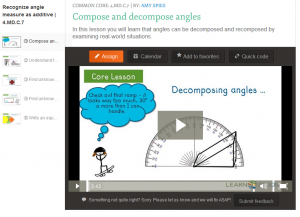# Measure and Sketch Angles in Whole Number Degrees

##Measurement & Data – 4th Grade

#### Geometric measurement: understand concepts of angle and measure angles.

CCSS.Math.Content.4.MD.C.6
Measure angles in whole-number degrees using a protractor. Sketch angles of specified measure.
CCSS.Math.Content.4.MD.C.7
Recognize angle measure as additive. When an angle is decomposed into non-overlapping parts, the angle measure of the whole is the sum of the angle measures of the parts. Solve addition and subtraction problems to find unknown angles on a diagram in real world and mathematical problems, e.g., by using an equation with a symbol for the unknown angle measure.

Teacher Notes
Connecting angles to real life an explaining how angles are important is very helpful for a students understanding.  A the angle of a ramp is an excellent example to explore.  Students need time to get comfortable with the tools (protractor most often) one can use to draw specific angle.
Connecting fractions and decomposing with angles into parts will lead students to being able to find an unknown angle.

Student Knowledge Goals
I know an angle is the union of two rays with the same initial point.
I know angles are measured with reference to a circle with its center at a common endpoint of the rays.
I know the unit of measure for angles is degrees and can be measured using a protractor.
I know a full rotation from the center of a circle is 360 degrees.
I know that "one degree" angle can be used to measure angles.
I know an angle that turns counterclockwise through "n" one-degree angles has a measure of "n" degrees.
I can sketch a variety of angles of a specified measure.
I can measure angles in whole-number degrees using a protractor.
I know non-overlapping angle segments can be added to find the total sum of the angle measures.
I know angle measures are additive.
I know the whole angle is the sum of the angle parts.
I know that angles can be decomposed into parts.
I can develop mental images for important benchmark angles (30o, 45o, 60o, and 90o).
I can determine whether to add or subtract to find the unknown angle on a diagram in real world and mathematical problems.
I can write an equation with a symbol for the unknown angle measure.

Vocabulary
acute angle
angle
complementary angles
decomposed
degrees
non-overlapping
obtuse angle
ray
right angle
straight angle
supplementary angles

Lessons
Engage NY Module 3 B-7 – Measure and draw angles.  Sketch given angle measures and verify with a protractor.
Engage NY Module 3 B-8 – Identify and measure angles as turns and recognize them in various contexts.
Engage NY Module 3 C-9 – Decompose angles using pattern blocks.
Engage NY Module 3 C-10 – Use the addition of adjacent angle measures to solve problems using a symbol for the unknown angle measure.
Engage NY Module 3 C-11 – Use the addition of adjacent angle measures to solve problems using a symbol for the unknown angle measure.

Online Problems and Assessments
Khan Academy – Questions and Video Lessons
Measure angles with a protractor
Estimate angle measurements